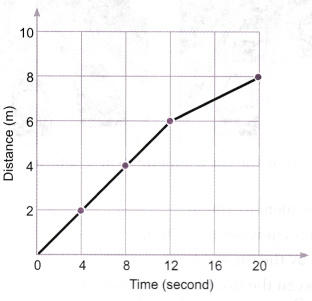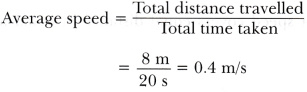# Given below as figure is the distance-time graph of the motion of an object

Given below as figure is the distance-time graph of the motion of an object.1. What will be the position of the object at 20 s?
2. What will be the distance travelled by the object in 12 s?
3. What is the average speed of the object?